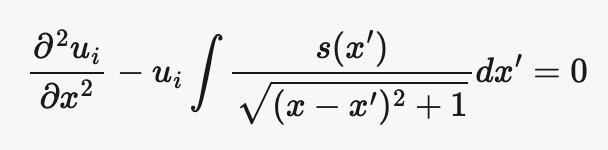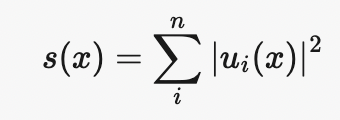# Handling an integral term in the differential equation

Hello, I am trying to solve a PDE where one of the terms is an integral of the solution variable. What would be the best way to calculate it?

Details:
We have multiple pdes of the form$$\frac{\partial^2 u_i}{\partial x^2} - u_i\int \frac{s(x’)}{\sqrt{(x-x’)^2+1}}dx’ = 0$$
Where $s$ is$$s(x) = \sum_i^n|u_i(x)|^2$$
The challenge here is that $s$ dependes on multiple $u_i$ and each solution $u_i$ depends on solving an integral of $s$ over the domain. What would be the best way to represent this in Modulus?

Some things that I have tried:
I tried using the Integral function in sympy (Integrals - SymPy 1.11 documentation)

#assume dom is already set
# Equation set up in modulus
x = Symbol('x')
t = Symbol('t')

# make input variables
input_variables = {'x':x, 't':t}

# make u_i list
# List of sympy functions (u0, u1, ..., un)
u_list = [Function('u%d' % i)(*input_variables) for i in range(n)]

# make s
amp_list = [u_list[i]**2  for i in range(n)]
s = sum(amp_list)

# Integral term
xp = Symbol('xp')
s_p = s.subs(x,xp)
s_integral = integrate(s_p/(sqrt((x-xp)**2 + 1)),( xp,-dom,dom))

# set equations
self.equations = {}

for i in range(n):
u = u_list[i]
self.equations['u%d' %i] = u.diff(x,2) - u * s_integral



Here, I get an error saying that Integral is not defined

...
...
File "/home/env/pinn/lib/python3.9/site-packages/modulus-22.3-py3.9.egg/modulus/sympy_utils/torch_printer.py", line 259, in forward
return {self.name: self.torch_expr(args)}
File "<lambdifygenerated-1>", line 5, in _lambdifygenerated
NameError: name 'Integral' is not defined


I checked the modulus sympy to torch converting functions and noticed that sympy.integrate is not in the list of functions that are converted to torch by modulus. This file is modulus/sympy_utils/torch_printer.py. I tried writing my own convertor to calculate an integral in pytorch from sympy but can’t figure that out.

I would be happy to explain this in more detail, and make necessary modifications in Modulus myself if you have some advice.

Hi had the same issue. When you try to write a PDE where the inputs depend on the solution, the Modulus is unable to unroll the computational graph. I fixed this in my own implementation.

Hi:

Did you find a solution?
My equation is much simpler: u’’ + u + Integral_of_u(from -1, to 1) = 0
but I cannot find a condition or implementation inside MODULUS to solve this type of equation.

Regards

Unfortunately we do not have native support integral terms inside of PDEs. The closest thing we have is the IntegralBoundaryConstraint but this is for cases where the entire equation is an integral.

You would likely need to implement a custom constraint or perhaps a custom node, there are multiple paths. You will need to approximate the integral by sampling points between [-1,1] which could be independent from the ones used to calculate the derivatives.

For the brute force custom constraint approach, I would suggest looking at the turbulent super resolution problem.